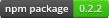Need Private Modules

# npm

## rng

0.2.2 • Public • Published

# Random## API

### Class: `RNG()`

Base class using `Math.random()` as PRNG. All other classes inherit from `RNG()`.

#### Methods

Number `next()` Get a random byte [0,255]

Number `random()` Same as `uniform()`, just to be compatible with the `Math.random()` style API

Number `uniform()` Get a uniform random number between 0 and 1

Number `normal()` Get normally distributed number, with a mean 0, variance 1

Number `range( min, max )` Get random integer in range [min,max]

Number `exp()` Get exponentionally distributed number with lambda 1

Number `poisson( mean )` Get poisson distributed number, the mean defaulting to 1

Number `gaussian( a )` Get gamma distributed number, using uniform, normal and exp with the Marsaglia-Tsang method

## Algorithms

### Class: `RNG.XOR( x, y, z, w )` - XOR Shift

#### Not (yet?) implemented:

• ISAAC - ISAAC (indirection, shift, accumulate, add, count)
• MWC - Multiply-With-Carry
• CMWC - Complementary-Multiply-With-Carry
• LCG - Linear Congruential Generator
• ICG - Inversive Congruential Generator
• LFG - Lagged Fibonacci Generator
• LFSR - Linear Feedback Shift Register
• NRPF - Naor-Reingold Pseudorandom Function
• BBS - Blum Blum Shub

## Keywords

none

### Install

`npm i rng`

### Repository

github.com/jhermsmeier/rng.js

### Homepage

github.com/jhermsmeier/rng.js